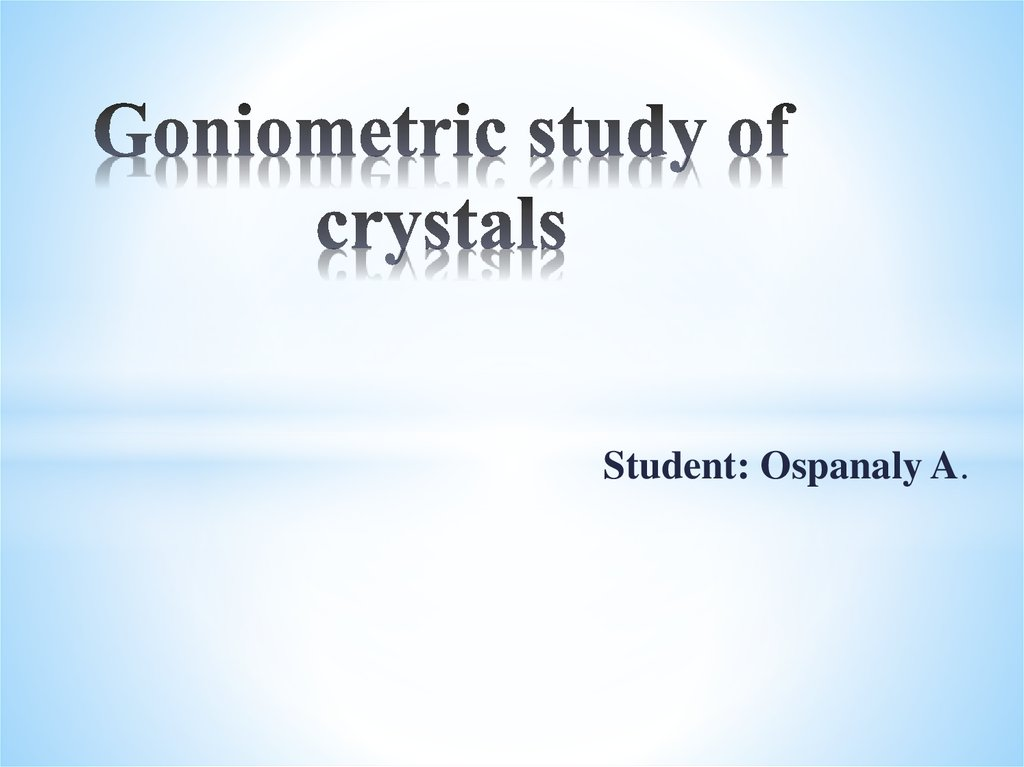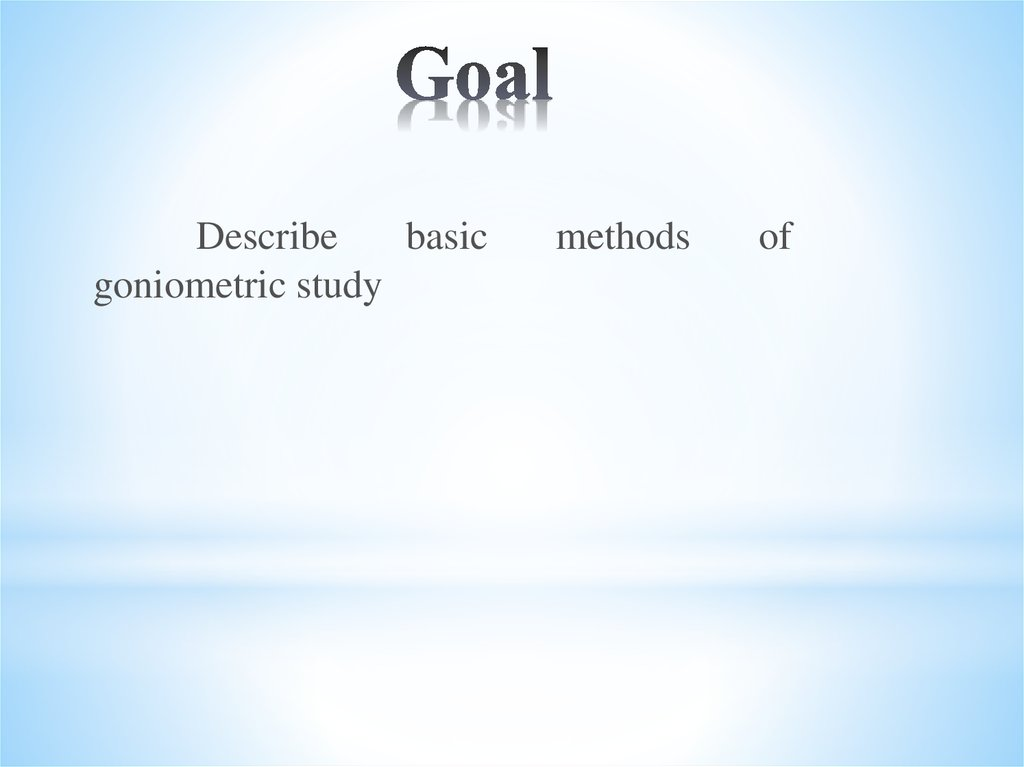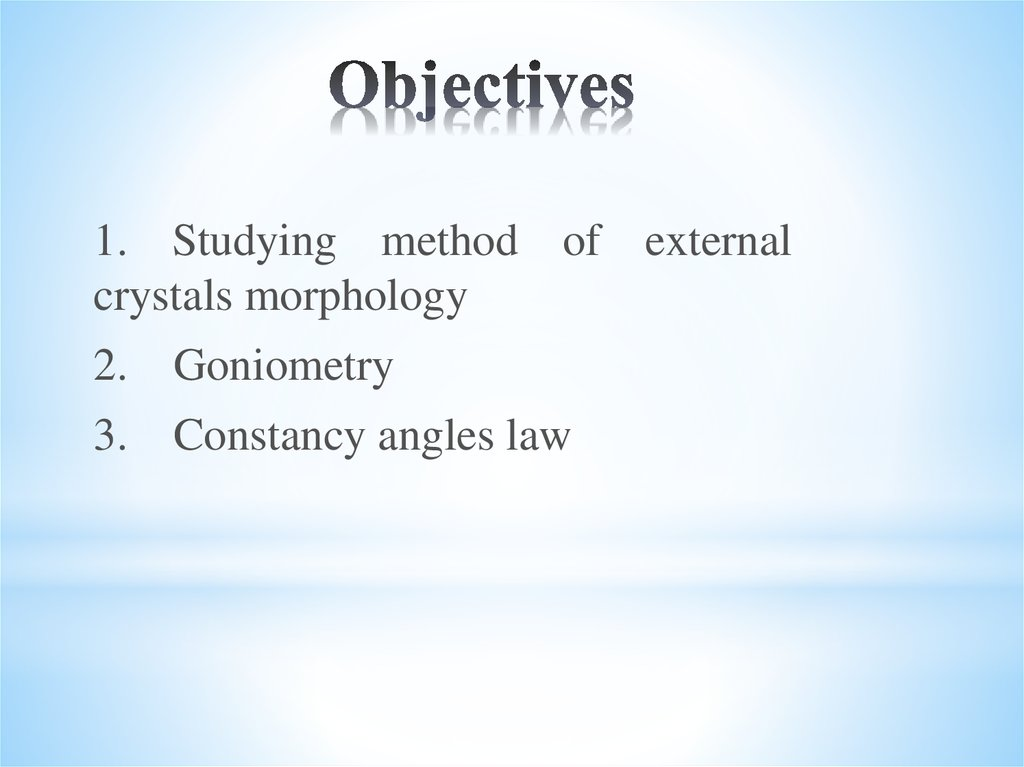# Goniometric study of crystals

## 1. Goniometric study of crystals

Student: Ospanaly A.

## 2. Goal

Describe
basic
goniometric study
methods
of

## 3. Objectives

1. Studying method of external
crystals morphology
2. Goniometry
3. Constancy angles law

## 4. Studying method of external crystals morphology

Crystal cut is an important characteristic that often
makes it possible to diagnose a substance without resorting to
additional analysis types. First studying method of external
crystals morphology was goniometry method, based on constant
angles law between corresponding faces. According to this law,
regardless of the same substance crystals variety , angles
between corresponding edges remain constant. For
measurement using applied and reflective goniometers. Despite
fact that this type’s first device was designed in the XVIII
century, such studies conduct in our time is very relevant, in
particular, to establish relationship between conditions for
obtaining crystals and their external form.

## 5.

Sufficiently large samples (more than
0.5 cm) with uneven and matte edges are
measured using applied goniometers.
Simplest application goniometer is a
protractor with a movable ruler. The crystal
is fixed in such a way that a pair of faces
between which the angle is measured tightly
clamped between protractor’s lower bar and
ruler. Double scale on the protractor allows
determining with sufficiently low accuracy
(about 0.5 °) both the angles between the
normals to the faces, counting readings from
left to right, and true intergeneral angles,
moving along scale from right to left.

## 6.

For more accurate
measurements (from a few
seconds to 1 min) and work
with small crystals with shiny
edges, reflective goniometers
(single and double circles) have
been developed, using good
reflectivity of high-quality
crystal surfaces and geometric
principles of incident
propagation and reflected light
rays.

## 7. Goniometry

Crystallography dealing section with angles
measurement is called goniometry (from the Greek word
“gony” - angle). A device for measuring angles between
crystal faces is called a goniometer. There are two types of
goniometers — applied and reflective. An applied
goniometer (Fig. 3) was proposed by Karanjo in 1780.
Measuring angles accuracy is 0.5 °. An applied
goniometer is usually used to measure crystals with face
sizes greater than 0.5 cm.. Reflective goniometers are
used to study small crystals with shiny edges and for more
accurate measurements.

## 8.

Figure 3. Applied
goniometer: 1rotating ruler, 2 measured crystal, 3 protractor
Figure 4. Diagram of
the reflective
goniometer:
1 - collimator, 2 telescope, 3 - crystal

## 9.

Reflective goniometer works as following sсheme
(Fig. 4). A narrow beam of rays is passed through the
collimator 1, equipped with a narrow slit and a collecting
lens, and falls on a crystal’s face (3). Reflected from it,
the rays beam hits the telescope (2). The reflection of the
light beam (“signal”) in the telescope can be obtained
only in the case where the angles of AOC and COB are
equal. By fixing the collimator position and optic tubes,
we rotate the crystal around the axis O until the face b
takes the position b of face a, and by fixing the
values ​between the first and second circle positions on
the axis of which the crystal is fixed, we can determine
the angle between normals C and D to edges a and b.

## 10.

Reflective goniometers allow measuring
angles with an accuracy of 1 or 30.
To study the geometric crystals shapes
used stereographic projections. To construct a
stereographic projection of a crystal from its
center O, mentally arbitrary radii describe a ball,
called a ball of projections. Then the diametrical
plane P of this ball (the projection plane) and the
diameter SS1 (axis of the projections)
perpendicular to it are carried out. Point S is
called point of view.

## 11.

To get a stereographic projection of any direction OA, its
output on the ball is connected with a straight line S point. The
trace of SA sight line on projections plane, that is point will be
the stereographic projection of this direction OA (Fig.5, a). To
obtain a plane stereographic projection (for example, ABCD), it is
transferred parallel to itself in the projections center and continued
until it intersects with the projection ball surface. As a result of
the intersection, a large circle arc ABCD is obtained on a ball, all
points of which are connected by straight lines with a view point
and receive a projecting cone. The resulting curve ABCD, in
which the projecting cone intersects with the projection plane, is
the stereographic projection of this plane ABCD (Fig.5, b). Thus,
the planes stereographic projections are represented by circular
arcs and the directions projections are shown by dots.

## 12. Constancy angles law

A single crystalline substance’s different forms
are subject to the same crystal structure. And since the
external form of given substance crystals is determined
by its internal structure, there must be a definite
regularity between the crystals cut elements of this
substance various forms. This pattern is expressed in
the constancy angles law .
The law says: The dihedral angles between the
respective crystal faces of the same substance at
constant temperature and pressure are constant.

## 13.

Relatively identical conditions reservation is
necessary, since different pressures and temperatures,
varying the interatomic distances in different directions,
cause angular values oscillations. In practice, these
oscillations are insignificant and can be disregarded. This
is true only for crystals with the same structure. In
connection with changes in physicochemical conditions,
radical reorganizations of the structure itself can occur, as a
result of which, for example, a diamond is transformed into
graphite (or vice versa). It goes without saying that the law
of constancy angles applies only to crystals belonging to
the same polymorphic modification. From this law it
follows that in most cases, by measuring angles, it is
possible to prove that the investigated crystal belongs to a
specific substance.

## 14.

Figure shows diamond
crystals of a regular and
distorted
shape,
the
octahedral faces of which
form the same angle
a = (109 ° 28 ').
The constancy of the angles
between the faces of diamond
crystals (crystals of regular and
distorted shapes)

## 15. Conclusion

Method of external
crystals
morphology was goniometry method,
based on constant angles law between
corresponding faces. According to this law,
regardless of the same substance crystals
variety , angles between corresponding
edges remain constant. For measurement
using applied and reflective goniometers.

## 16. VOCABULARY

*external– [ikˈstərnl] – внешний - сыртқы
*measure– [ˈmeZHər] – измерения өлшем
*dihedral–[dīˈhēdrəl] – двугранныйекіқырлы
*beam– [bēm] – луч - сәуле

Thanks for your
attention!